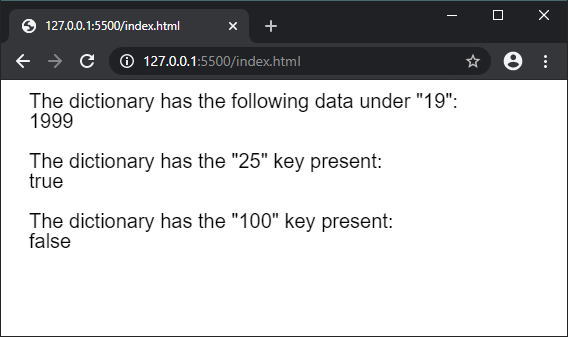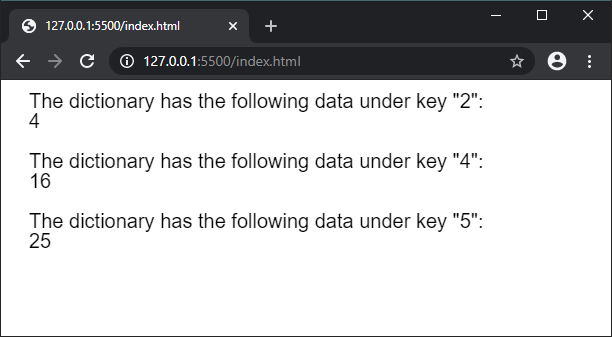# p5.js | createNumberDict() Function

• Last Updated : 20 Feb, 2020

The createNumberDict() function is used to create a p5.NumberDict instance with the given data. The data can be individually passed a key-value pair or given as a collection of values using an object.

Syntax:

`createNumberDict(key, value)`

or

`createNumberDict(object)`

Parameters:

• key: It specifies the number that is used as the key in the dictionary.
• value: It specifies the number that is used as the value in the dictionary.
• object: It specifies the object that is used as the dictionary.

Return Value: It returns a p5.NumberDict object with the given data.

The program below illustrate the createNumberDict() function in p5.js:

Example 1:

 `function` `setup() { ` `  ``createCanvas(500, 200); ` `  ``textSize(20); ` ` `  `  ``// Creating a number dictionary ` `  ``// with the given key and value pair ` `  ``let mydict = createNumberDict(``"19"``, ``"1999"``); ` ` `  `  ``// Accessing the data using the data property ` `  ``text(``'The dictionary has the following data under "19":'``, 20, 20); ` `  ``text(mydict.data[``"19"``], 20, 40); ` ` `  `  ``// Checking if a key exists in the dictionary ` `  ``text(``'The dictionary has the "25" key present:'``, 20, 80); ` `  ``text(mydict.hasKey(``"19"``), 20, 100); ` ` `  `  ``text(``'The dictionary has the "100" key present:'``, 20, 140); ` `  ``text(mydict.hasKey(``"100"``), 20, 160); ` `} `

Output:Example 2:

 `function` `setup() { ` `  ``createCanvas(600, 200); ` `  ``textSize(20); ` ` `  `  ``let squaresObject = { ` `    ``2: 4, ` `    ``3: 9, ` `    ``4: 16, ` `    ``5: 25 ` `  ``} ` ` `  `  ``// Creating a number dictionary ` `  ``// with the above object ` `  ``let mydict = createNumberDict(squaresObject); ` ` `  `  ``// Accessing the data using the data property ` `  ``text(``'The dictionary has the following data under key "2":'``, 20, 20); ` `  ``text(mydict.data[``"2"``], 20, 40); ` ` `  `  ``text(``'The dictionary has the following data under key "4":'``, 20, 80); ` `  ``text(mydict.data[``"4"``], 20, 100); ` ` `  `  ``text(``'The dictionary has the following data under key "5":'``, 20, 140); ` `  ``text(mydict.data[``"5"``], 20, 160); ` `} `

Output:Online editor: https://editor.p5js.org/

My Personal Notes arrow_drop_up
Recommended Articles
Page :